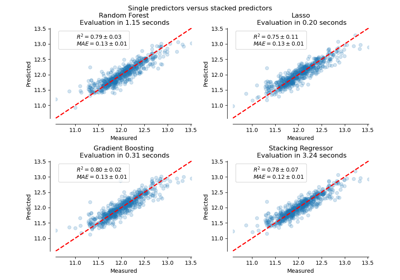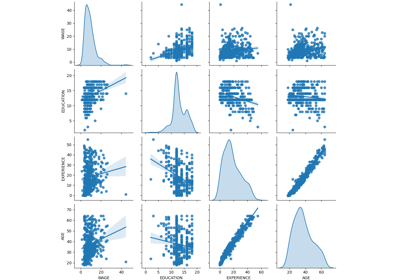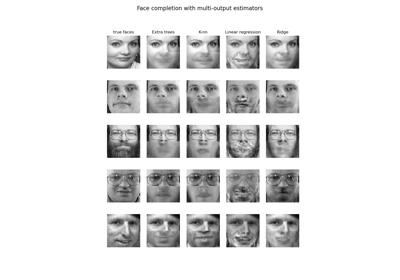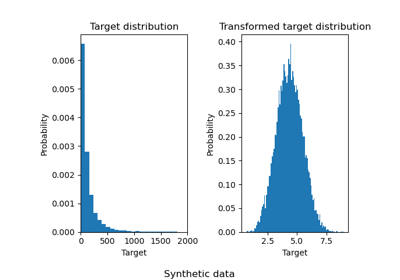# 3.2.4.1.9. sklearn.linear_model.RidgeCV¶

class sklearn.linear_model.RidgeCV(alphas=(0.1, 1.0, 10.0), *, fit_intercept=True, normalize=False, scoring=None, cv=None, gcv_mode=None, store_cv_values=False)[source]

Ridge regression with built-in cross-validation.

See glossary entry for cross-validation estimator.

By default, it performs Generalized Cross-Validation, which is a form of efficient Leave-One-Out cross-validation.

Read more in the User Guide.

Parameters
alphasndarray of shape (n_alphas,), default=(0.1, 1.0, 10.0)

Array of alpha values to try. Regularization strength; must be a positive float. Regularization improves the conditioning of the problem and reduces the variance of the estimates. Larger values specify stronger regularization. Alpha corresponds to 1 / (2C) in other linear models such as LogisticRegression or sklearn.svm.LinearSVC. If using generalized cross-validation, alphas must be positive.

fit_interceptbool, default=True

Whether to calculate the intercept for this model. If set to false, no intercept will be used in calculations (i.e. data is expected to be centered).

normalizebool, default=False

This parameter is ignored when fit_intercept is set to False. If True, the regressors X will be normalized before regression by subtracting the mean and dividing by the l2-norm. If you wish to standardize, please use sklearn.preprocessing.StandardScaler before calling fit on an estimator with normalize=False.

scoringstring, callable, default=None

A string (see model evaluation documentation) or a scorer callable object / function with signature scorer(estimator, X, y). If None, the negative mean squared error if cv is ‘auto’ or None (i.e. when using generalized cross-validation), and r2 score otherwise.

cvint, cross-validation generator or an iterable, default=None

Determines the cross-validation splitting strategy. Possible inputs for cv are:

• None, to use the efficient Leave-One-Out cross-validation (also known as Generalized Cross-Validation).

• integer, to specify the number of folds.

• An iterable yielding (train, test) splits as arrays of indices.

For integer/None inputs, if y is binary or multiclass, sklearn.model_selection.StratifiedKFold is used, else, sklearn.model_selection.KFold is used.

Refer User Guide for the various cross-validation strategies that can be used here.

gcv_mode{‘auto’, ‘svd’, eigen’}, default=’auto’

Flag indicating which strategy to use when performing Generalized Cross-Validation. Options are:

'auto' : use 'svd' if n_samples > n_features, otherwise use 'eigen'
'svd' : force use of singular value decomposition of X when X is
dense, eigenvalue decomposition of X^T.X when X is sparse.
'eigen' : force computation via eigendecomposition of X.X^T


The ‘auto’ mode is the default and is intended to pick the cheaper option of the two depending on the shape of the training data.

store_cv_valuesbool, default=False

Flag indicating if the cross-validation values corresponding to each alpha should be stored in the cv_values_ attribute (see below). This flag is only compatible with cv=None (i.e. using Generalized Cross-Validation).

Attributes
cv_values_ndarray of shape (n_samples, n_alphas) or shape (n_samples, n_targets, n_alphas), optional

Cross-validation values for each alpha (only available if store_cv_values=True and cv=None). After fit() has been called, this attribute will contain the mean squared errors (by default) or the values of the {loss,score}_func function (if provided in the constructor).

coef_ndarray of shape (n_features) or (n_targets, n_features)

Weight vector(s).

intercept_float or ndarray of shape (n_targets,)

Independent term in decision function. Set to 0.0 if fit_intercept = False.

alpha_float

Estimated regularization parameter.

best_score_float

Score of base estimator with best alpha.

Ridge

Ridge regression

RidgeClassifier

Ridge classifier

RidgeClassifierCV

Ridge classifier with built-in cross validation

Examples

>>> from sklearn.datasets import load_diabetes
>>> from sklearn.linear_model import RidgeCV
>>> clf = RidgeCV(alphas=[1e-3, 1e-2, 1e-1, 1]).fit(X, y)
>>> clf.score(X, y)
0.5166...


Methods

 fit(X, y[, sample_weight]) Fit Ridge regression model with cv. get_params([deep]) Get parameters for this estimator. Predict using the linear model. score(X, y[, sample_weight]) Return the coefficient of determination R^2 of the prediction. set_params(**params) Set the parameters of this estimator.
__init__(alphas=(0.1, 1.0, 10.0), *, fit_intercept=True, normalize=False, scoring=None, cv=None, gcv_mode=None, store_cv_values=False)[source]

Initialize self. See help(type(self)) for accurate signature.

fit(X, y, sample_weight=None)[source]

Fit Ridge regression model with cv.

Parameters
Xndarray of shape (n_samples, n_features)

Training data. If using GCV, will be cast to float64 if necessary.

yndarray of shape (n_samples,) or (n_samples, n_targets)

Target values. Will be cast to X’s dtype if necessary.

sample_weightfloat or ndarray of shape (n_samples,), default=None

Individual weights for each sample. If given a float, every sample will have the same weight.

Returns
selfobject

Notes

When sample_weight is provided, the selected hyperparameter may depend on whether we use generalized cross-validation (cv=None or cv=’auto’) or another form of cross-validation, because only generalized cross-validation takes the sample weights into account when computing the validation score.

get_params(deep=True)[source]

Get parameters for this estimator.

Parameters
deepbool, default=True

If True, will return the parameters for this estimator and contained subobjects that are estimators.

Returns
paramsmapping of string to any

Parameter names mapped to their values.

predict(X)[source]

Predict using the linear model.

Parameters
Xarray_like or sparse matrix, shape (n_samples, n_features)

Samples.

Returns
Carray, shape (n_samples,)

Returns predicted values.

score(X, y, sample_weight=None)[source]

Return the coefficient of determination R^2 of the prediction.

The coefficient R^2 is defined as (1 - u/v), where u is the residual sum of squares ((y_true - y_pred) ** 2).sum() and v is the total sum of squares ((y_true - y_true.mean()) ** 2).sum(). The best possible score is 1.0 and it can be negative (because the model can be arbitrarily worse). A constant model that always predicts the expected value of y, disregarding the input features, would get a R^2 score of 0.0.

Parameters
Xarray-like of shape (n_samples, n_features)

Test samples. For some estimators this may be a precomputed kernel matrix or a list of generic objects instead, shape = (n_samples, n_samples_fitted), where n_samples_fitted is the number of samples used in the fitting for the estimator.

yarray-like of shape (n_samples,) or (n_samples, n_outputs)

True values for X.

sample_weightarray-like of shape (n_samples,), default=None

Sample weights.

Returns
scorefloat

R^2 of self.predict(X) wrt. y.

Notes

The R2 score used when calling score on a regressor uses multioutput='uniform_average' from version 0.23 to keep consistent with default value of r2_score. This influences the score method of all the multioutput regressors (except for MultiOutputRegressor).

set_params(**params)[source]

Set the parameters of this estimator.

The method works on simple estimators as well as on nested objects (such as pipelines). The latter have parameters of the form <component>__<parameter> so that it’s possible to update each component of a nested object.

Parameters
**paramsdict

Estimator parameters.

Returns
selfobject

Estimator instance.

## 3.2.4.1.9.1. Examples using sklearn.linear_model.RidgeCV¶